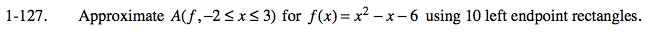### Home > CALC > Chapter 1 > Lesson 1.3.3 > Problem1-127

1-127.

Approximate A(f, −2 ≤ x ≤ 3) for f(x) = x2x − 6 using 10 left endpoint rectangles. 1-127 HW eTool (Desmos). Homework Help ✎Find the sum of the areas of all 10 rectangles. What is the width of each rectangle? How can you calculate the height of each one?

Use the eTool below to view the graph.
Click the link at right for the full version of the eTool: Calc 1-127 HW eTool